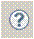# Viewing and Editing Structured Proofs

```Contents
Viewing proofs
Editing proofs
```

## Viewing Proofs

When you open in the module editor a module containing a theorem wth a proof, you will see a  (-)  next to the theorem and next to each step that has a subproof.  To avoid repeatedly saying step or theorem, we here take a step to be either a theorem or a step of a theorem's proof.  Clicking on the  (-)  next to a step hides the step's proof and changes the  (-)  to a  (+) . Clicking on the  (+)  undoes the effect of clicking on the  (-) .

Right-clicking on a step provides a menu with a section containing the following commands for hiding and revealing parts of the proof of the step at which the cursor is positioned.  These commands can also be executed with the indicated pair of keystrokes.  If you just type Ctl+G (the first keystroke) and wait a second, you will see a list of all the commands telling you what keystroke you should type next.

Show Current Subtree     Ctl+G Ctl+S
Reveals the proof of the step.

Hide Current Subtree     Ctl+G Ctl+H
Hides the proof of the step.

Show Children Only     Ctl+G Ctl+C
Reveals the top level of the step's proof.

Focus on Step     Ctl+G Ctl+F
Hides everything except the top level of the step's proof and the siblings (steps at the same level as) of the step and of every ancestor of that step in the proof.
The following commands can be performed by right-clicking anywhere in the module.
Show All Proofs     Ctl+G Ctl+A
Reveals the complete proof of every theorem in the module.

Hide All Proofs     Ctl+G Ctl+N
Hides the proof of every theorem in the module.
The Focus on Step command performed outside a proof is equivalent to Hide All Proofs

## Editing Proofs

There are two commands especially for editing proofs:
Decompose Proof     Ctl+G Ctl+D
To use this command, put the cursor in a theorem or proof step that has either no proof or else a leaf proof (a `BY` or `OBVIOUS` proof) that TLAPS can't verify.  This command raises a dialog window that allows you to decompose the proof of the theorem or step into simpler steps.  The dialog contains abutton that raises this help page, which explains what you can do with this dialog.

Renumber Proof     Ctl+G Ctl+R
A proof step is either named or unnamed.  An unnamed step begins with just a level number, like `<4>`.  A named step begins with a level number and a name, like `<4>7` or `<4>xy2` (optionally followed by a period).  Putting the cursor on a proof step and executing the Renumber Proof command causes all the named top-level steps of its proof to be renamed with consecutive numbers, like `<4>1`, `<4>2`, etc.  However, you might want to use some meaningful step names like `<2>NextImpliesInv1` that you don't want to be turned into numbers.  A module editor preference provides options to control what step names don't get turned into numbers.  There is also a preference that determines whether the module should be saved after executing the command.
The Toolbox editor's block-selection mode can help in formatting proofs.  We would like to create additional editor commands to make it easier to write and edit proofs, but we don't know what commands would be useful.  Please tell us what commands you would like.

↑ Proofs